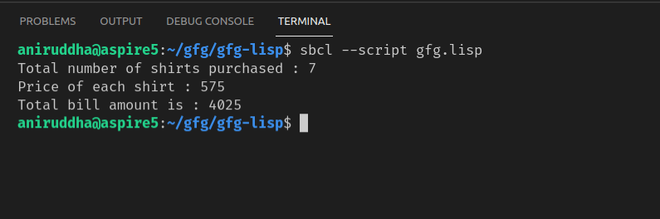Constants in LISP

• Last Updated : 16 Oct, 2021

In LISP all constants are global variables. Values of constant never change throughout the execution of the program.

Defining constant in LISP:

New global constants are defined using the DEFCONSTANT construct
Syntax:

(defconstant name initial-value-form
"documentation-string")

Example:

Let’s create a global constant whose value will contain the rate of shirt

(defconstant shirt-rate 575
"shirt-rate is having a constant value of 575")

Now let’s create a function that will calculate the total bill by taking the number of shirts as input and then multiplying it with our previously defined constant shirt-rate

(defun calc-total-bill (n)
"Calculates the total bill by multiplying the number of shirts purchased with its constant rate"
(* shirt-rate n))

Let’s call this function

(format t "Total number of shirts purchased : 7~%")
(format t "Price of each shirt : ~2d~%" shirt-rate)
(format t "Total bill amount is : ~2d~%" (calc-total-bill 7))

Code:

Lisp

 (defconstant shirt-rate 575  "shirt-rate is having a constant value of 575")  (defun calc-total-bill (n)  "Calculates the total bill by multiplying the number of   shirts purchased with its constant rate"  (* shirt-rate n))  (format t "Total number of shirts purchased : 7~%")(format t "Price of each shirt : ~2d~%" shirt-rate)(format t "Total bill amount is : ~2d~%" (calc-total-bill 7))

Output :We can check if some symbol is the name of a global variable or constant by using boundp predicate, As it is the predicate it returns if it finds the constant with a particular name and will return NIL if it doesn’t.

For example, in our program, we have a constant shirt-rate defined so let’s run this

Lisp

 (write (boundp 'shirt-rate))(terpri)(write (boundp 'rate))

Output :

T
NIL

As expected it has returned T when it finds a constant shirt-rate but returns NIL when it can’t find the constant rate in the program.

My Personal Notes arrow_drop_up